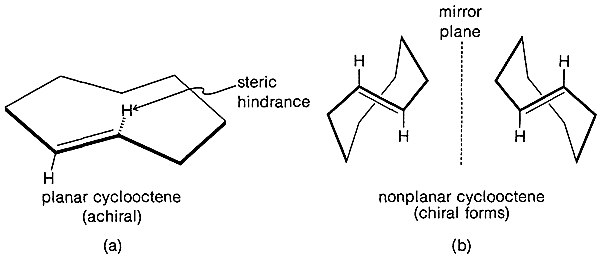# 12.7: Cycloalkenes and Cycloalkanes

$$\newcommand{\vecs}{\overset { \rightharpoonup} {\mathbf{#1}} }$$ $$\newcommand{\vecd}{\overset{-\!-\!\rightharpoonup}{\vphantom{a}\smash {#1}}}$$$$\newcommand{\id}{\mathrm{id}}$$ $$\newcommand{\Span}{\mathrm{span}}$$ $$\newcommand{\kernel}{\mathrm{null}\,}$$ $$\newcommand{\range}{\mathrm{range}\,}$$ $$\newcommand{\RealPart}{\mathrm{Re}}$$ $$\newcommand{\ImaginaryPart}{\mathrm{Im}}$$ $$\newcommand{\Argument}{\mathrm{Arg}}$$ $$\newcommand{\norm}{\| #1 \|}$$ $$\newcommand{\inner}{\langle #1, #2 \rangle}$$ $$\newcommand{\Span}{\mathrm{span}}$$ $$\newcommand{\id}{\mathrm{id}}$$ $$\newcommand{\Span}{\mathrm{span}}$$ $$\newcommand{\kernel}{\mathrm{null}\,}$$ $$\newcommand{\range}{\mathrm{range}\,}$$ $$\newcommand{\RealPart}{\mathrm{Re}}$$ $$\newcommand{\ImaginaryPart}{\mathrm{Im}}$$ $$\newcommand{\Argument}{\mathrm{Arg}}$$ $$\newcommand{\norm}{\| #1 \|}$$ $$\newcommand{\inner}{\langle #1, #2 \rangle}$$ $$\newcommand{\Span}{\mathrm{span}}$$

The $$\ce{C-C=C}$$ angle in alkenes normally is about $$122^\text{o}$$, which is $$10^\text{o}$$ larger than the normal $$\ce{C-C-C}$$ angle in cycloalkanes. This means that we would expect about $$20^\text{o}$$ more angle strain in small-ring cycloalkenes than in the cycloalkanes with the same numbers of carbons in the ring. Comparison of the data for cycloalkenes in Table 12-5 and for cycloalkanes in Table 12-3 reveals that this expectation is realized for cyclopropene, but is less conspicuous for cyclobutene and cyclopentene. The reason for this is not clear, but may be connected in part with the $$\ce{C-H}$$ bond strengths (see Section 12-4B).

Cyclopropene has rather exceptional properties compared to the other cycloalkenes. It is quite unstable and the liquid polymerizes spontaneously although slowly, even at $$-80^\text{o}$$. This substance, unlike other alkenes, reacts rapidly with iodine and behaves like an alkyne in that one of its double-bond hydrogens is replaced in silver-ammonia solution to yield an alkynide-like silver complex.

Table 12-5: Properties of Some Cycloalkenes and CycloalkynesFigure 12-20b) are stable and thus resolvable.Figure 12-20 Representation of (a) achiral and (b) chiral conformations of trans-cycloalkenes, using trans-cyclooctene as a specific example. For trans-cyclooctene, the achiral state is highly strained because of interference between the “inside” alkenic hydrogen and the $$\ce{CH_2}$$ groups on the other side of the ring. Consequently the mirror-image forms are quite stable. With trans-cyclononene, the planar state is much less strained and, as a result, the optical isomers are much less stable. With trans-cyclodecene, it has not been possible to isolate mirror-image forms because the two forms corresponding to (b) are interconverted through achiral planar conformations corresponding to (a) about $$10^{16}$$ times faster than with trans-cyclooctene.

The $$\ce{C-C=C}$$ bond angles in alkynes normally are $$180^\text{o}$$ and the angle strain involved in making a small-ring cycloalkyne, such as cyclopropyne, apparently is prohibitive. The smallest reasonably stable member of the series is cyclooctyne, and its properties, along with those of some higher homologs, are shown in Table 12-5. Strong evidence has been adduced for the existence of cyclopentyne, cyclohexyne, and cycloheptyne as unstable reaction intermediates.